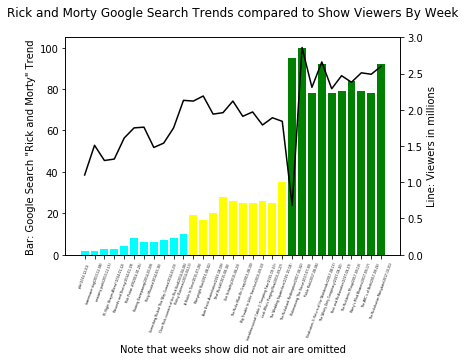In :
#https://en.wikipedia.org/wiki/List_of_Rick_and_Morty_episodes#Ratings
#google searches for the term "rick and morty" since the show aired on 12/2/13 to last episode released date of 10/1/17

import pandas as pd
import matplotlib.pyplot as plt
import numpy as np

df=df[df.episode_name.str.len().gt(4)]
df.episode_name=df.episode_name+'('+df.week+')'
df.season = df.season.map({1: 'cyan', 2: 'yellow',3:'green','':'black'})
df.season=df.season.astype(str)
df['log_viewers']=np.log(pd.to_numeric(df.viewers))

In :
#print(len(list(df.week))+1)
index= list(range(1,len(list(df.week))+1))#list(df.week)#
label = list(df.episode_name)#list(df.week)
colors=list(df.season)
f = plt.figure()
plt.bar(index, df.search_trend,color=colors)

plt.xlabel('Note that weeks show did not air are omitted')
plt.ylabel('Bar: Google Search "Rick and Morty" Trend')
plt.xticks(index, label, fontsize=3, rotation=70)

plt.title('Rick and Morty Google Search Trends compared to Show Viewers By Week\n')

y = df.viewers#df.log_viewers
axes2 = plt.twinx()
axes2.plot(index, y, color='k')
axes2.set_ylim(0, 3)
axes2.set_ylabel('Line: Viewers in millions')

plt.show()In :
f.savefig("/home/king/Downloads/rickandmorty4.pdf", bbox_inches='tight')